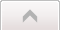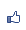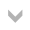Contents ...
udn網路城邦

2017/01/03 06:06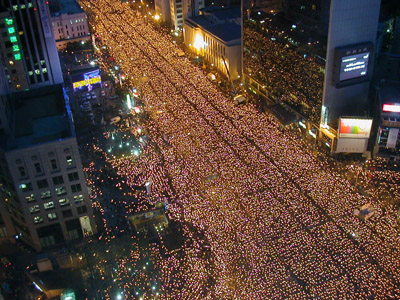2008年韓國民眾才為美牛問題進行大規模示威抗議不久，馬英九政府在美方壓力之下也簽署了「美國牛肉輸台議定書」，引起民眾一片譁然。政府希望能說服民眾的理由，是吃美國牛肉得狂牛病因而致命的機會極小。當時美國在臺協會台北辦事處處長司徒文說沒有人吃美國牛肉致死，而台灣在2008年有一千多人騎機車死亡，「沒有人說騎機車不安全」。衛生署長葉金川也附和說吃美牛得狂牛病的機會跟被雷打到兩次一樣，「是非常低的風險」。

2010年，我隨台灣旅美政治學者訪問團回台，有機會面見當時的行政院吳敦義院長及馬英九總統，談到美牛進口爭議。我建言說用「機率」來為食安政策辯護是無法說服民眾的。我說：政府發行公益彩券，其中獎機率幾乎為零，難道政府要勸民眾放棄中獎的希望？而即使中獎機率極小是科學的客觀事實，這對民眾也沒有說服力，因為民眾買彩劵，相信的是「命運」而不是「機會」。

「如果不覺得騎機車不安全，便沒有理由說吃美牛不安全」，這是錯誤的命題。首先，民眾對交通安全並不是完全放心。他們即使出門不見得會燒香禱告，也可能在身上、車上放平安符。而一般人在親友出遠門時，總會致上旅途平安的祝福，正是反映了對交通安全的顧慮。他們了解，意外事故是有可能降臨自身的，只是現代生活不能沒有交通，不能因咽廢食罷了。說不怕車禍便沒有理由怕美牛或核災食品，難道要民眾在上餐廳時攜帶平安符或在餐桌上互相祝禱“Have a safe meal”? 而且，在交通問題上，民眾期待的是政府能夠從基本建設上減少車禍的機率，而開放有食安顧慮的食品進口卻是增加致病的機率，這當然不符合民眾對政府的期待。

• 記憶所及簡法（availability heuristic）：以記憶所及的相關事件來估計機率
• 代表性簡法（representativeness heuristic）：根據事件的代表性來估計機率

• 「林教授自2010年以來，諸事不順。」
• 「林教授自2010年與馬英九總統握手以來，諸事不順。」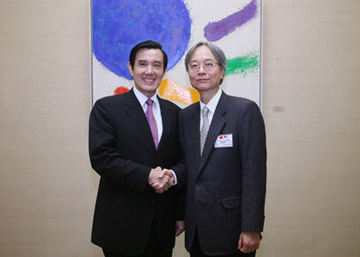• 客觀機率為0的結果其主觀機率亦為0：π(0)=0
• 客觀機率為1的結果期主觀機率亦為1：π(1)=1
• 客觀機率甚小的結果其主觀機率大於客觀機率：若ｐ甚小，則π(p)>p
• 客觀機率較大的結果其主觀機率小於客觀機率：若ｐ甚小，則π(1-p)<1-p。
• 較大客觀機率在主觀上被低估的幅度可能大於甚小客觀機率在主觀上被高估的程度：若ｐ甚小，則(1-p)-π(1-p)≥π(p)-p，也就是π(p)+π(1-p)≤1
• 其它與本文無直接關係的假設。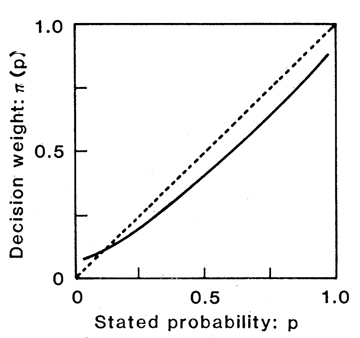• 無「得」無「失」的結果其價值為0：ｖ(0)=0
• 有所「得」的正面的結果，其價值函數往正向呈現邊際效益遞減的趨勢。
• 有所「失」的負面的結果，其價值函數往負向呈現邊際效益遞減的趨勢。
• 相對於同樣數量的「得」，「失」所造成的傷害程度大於「得」所帶來的滿足程度：若x<0，則|v(x)|>|v(-x)|
• 風險決策的預期價值為「得」、「失」結果價值用主觀機率加權後的總和：若風險決策得到x結果的客觀機率為p，得到y結果的客觀機率為q，則風險決策的預期價值為V(x,p;y,q)=π(p)v(x)+π(q)v(y)，此預期價值也稱作「前景」（prospect）。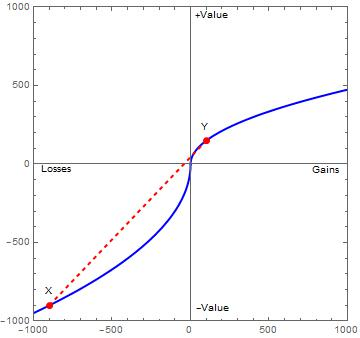1. 不支持開放進口，其結果為s。這個結果是確定的也就是客觀機率為1。若不支持開放進口並不會改變現狀，則我們可以假設現狀的價值為v(s)=0 。因為此結果的客觀機率為1，其主觀機率π(1)=1。選項Ａ的前景為0
2. 支持開放進口，其結果為x的客觀機率為ｐ、為y的客觀機率為q。選項Ｂ的前景為兩種結果用主觀機率加權後的預期價值V(x,p;y,q)=π(p)v(x)+π(q)v(y)

V(x,p;y,q)=π(p)v(x)+π(q)v(y) > 0

-v(x) < [π(q)/π(p)]v(y)

|v(x)| < [π(q)/π(p)]v(y)

|v(x)| < 90v(y) 或 |v(x)|/v(y) < 90

|v(x)| < 990v(y)

V(x,p;y,q) = π(p)v(x) + π(q)v(y) > v(s)

|v(x)| < [π(q)/π(p)]v(y) + [1/π(p)]|v(s)|

|v(x)| < 90v(y) + 100|v(s)|